# The 555 Fan

## 1 Using a potentiometer

### Learn It

• A potentiometer is a component we can use in our circuits as potential divider.
• Before you learn about the potentiometer, you need to learn what is meant by a potential divider.

### Try It

• Open Circuit Wizard and create the circuit shown below.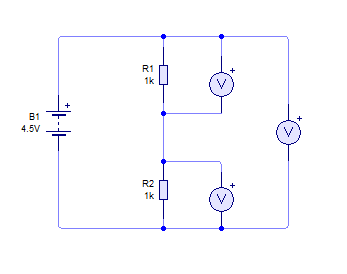• Now press play and the three voltmeters should show potential difference across each of the resistors and across the pair of them.
• Because both resistors have the same value, the potential difference supplies by the battery is equally divided across each resistor.
• Now let's change the value of one of the resistors.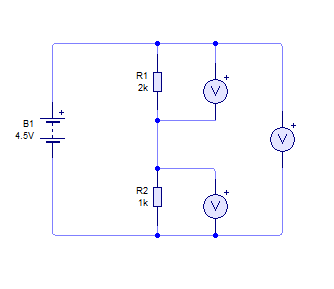• Press play again, and see how the potential differences have changed.

• Complete the table below by changing the values in your circuit diagram.
• Take a screenshot of each of your circuit results to upload to www.bournetolearn.com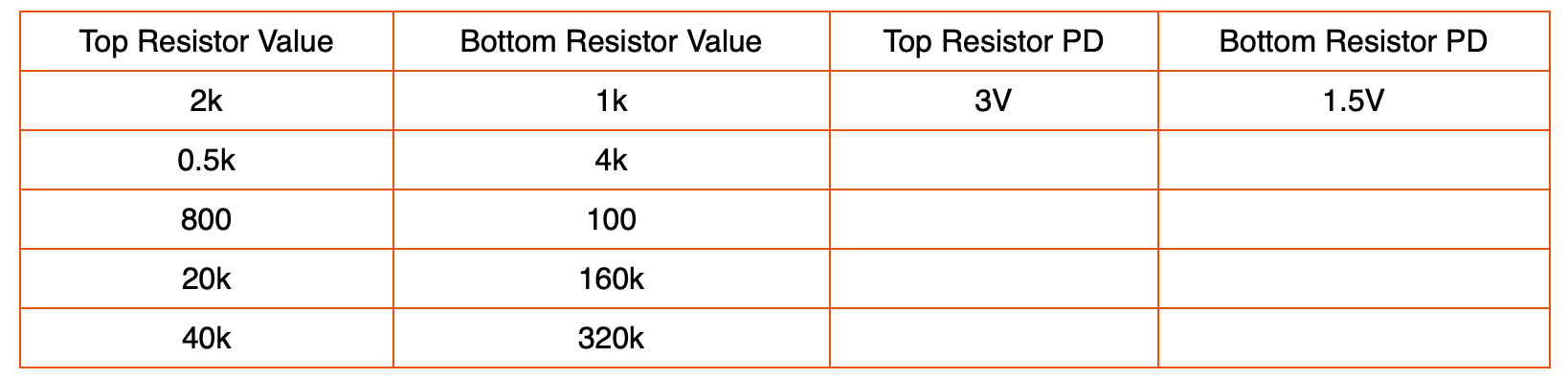• Can you see a pattern between resistor values and potential difference?
• You will also need to upload you screenshots of the circuits.

### Learn It

• If we know the resistor values and the total potential difference provided by the battery, it is easy to work out what the potential differences across each resistor will be.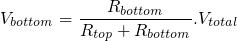• If we want to find Vtop then we can just do the following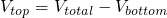### Try It

• Why is this important?
• By changing resistor values we can influence the timing of the 555 timer chip. This will speed up or slow down the time the capacitor takes to charge.
• Set up the circuit as shown below.• Simulate the circuit and then start adjusting the potentiometer.
• If you hover the arrow over the motor, you can see the rpm of the motor and the current flow.
• Change the value of the potentiometer and see how it affects the speed and current flow through the motor.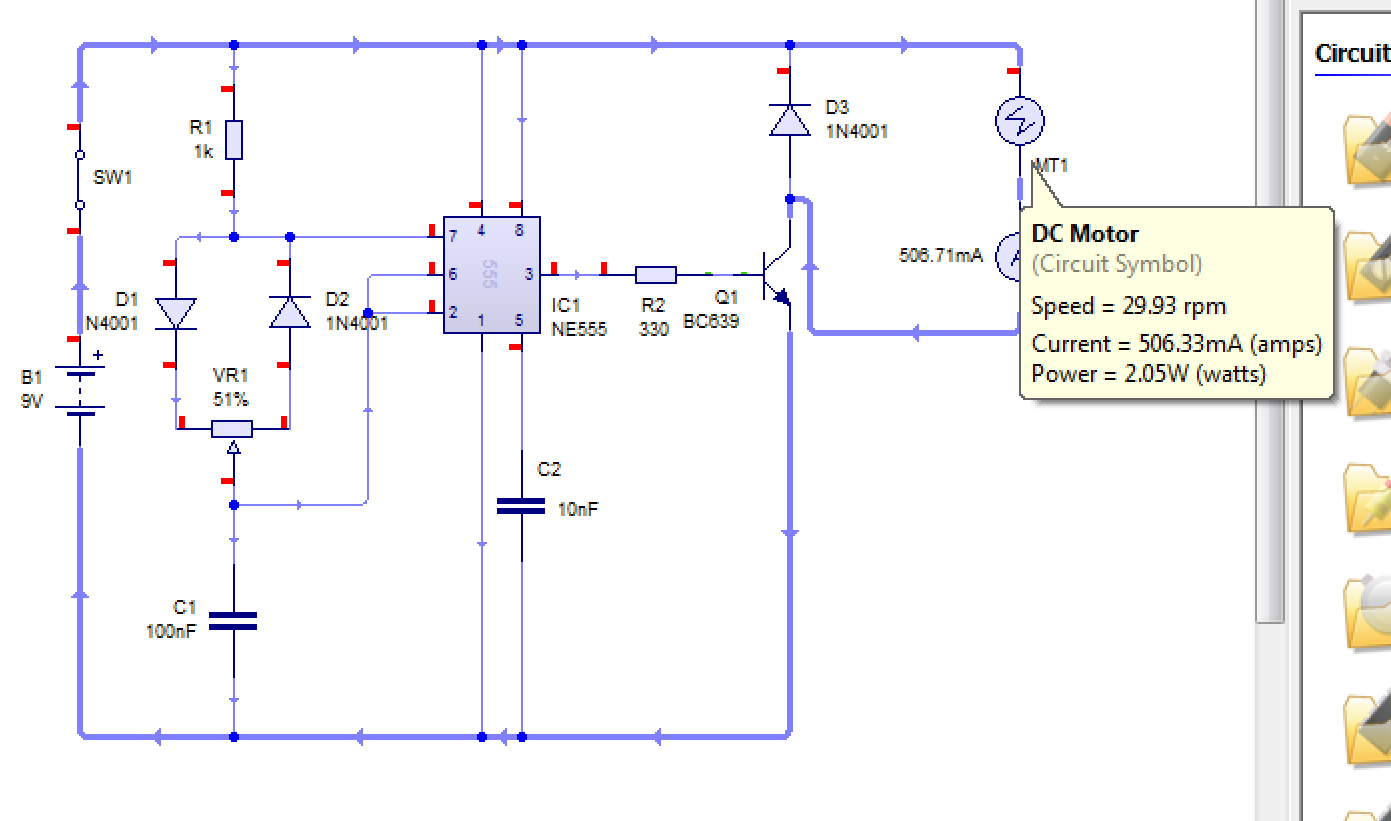• Draw a graph to display how changing the value of the potentiometer changes the speed of the motor.

### Learn It

• It would be inconvenient to have to swap in and out resistors to alter the potential difference reaching our 555 chip. This is where a potentiometer comes in handy.
• Imagine you had a length of resistive wire - as shown below.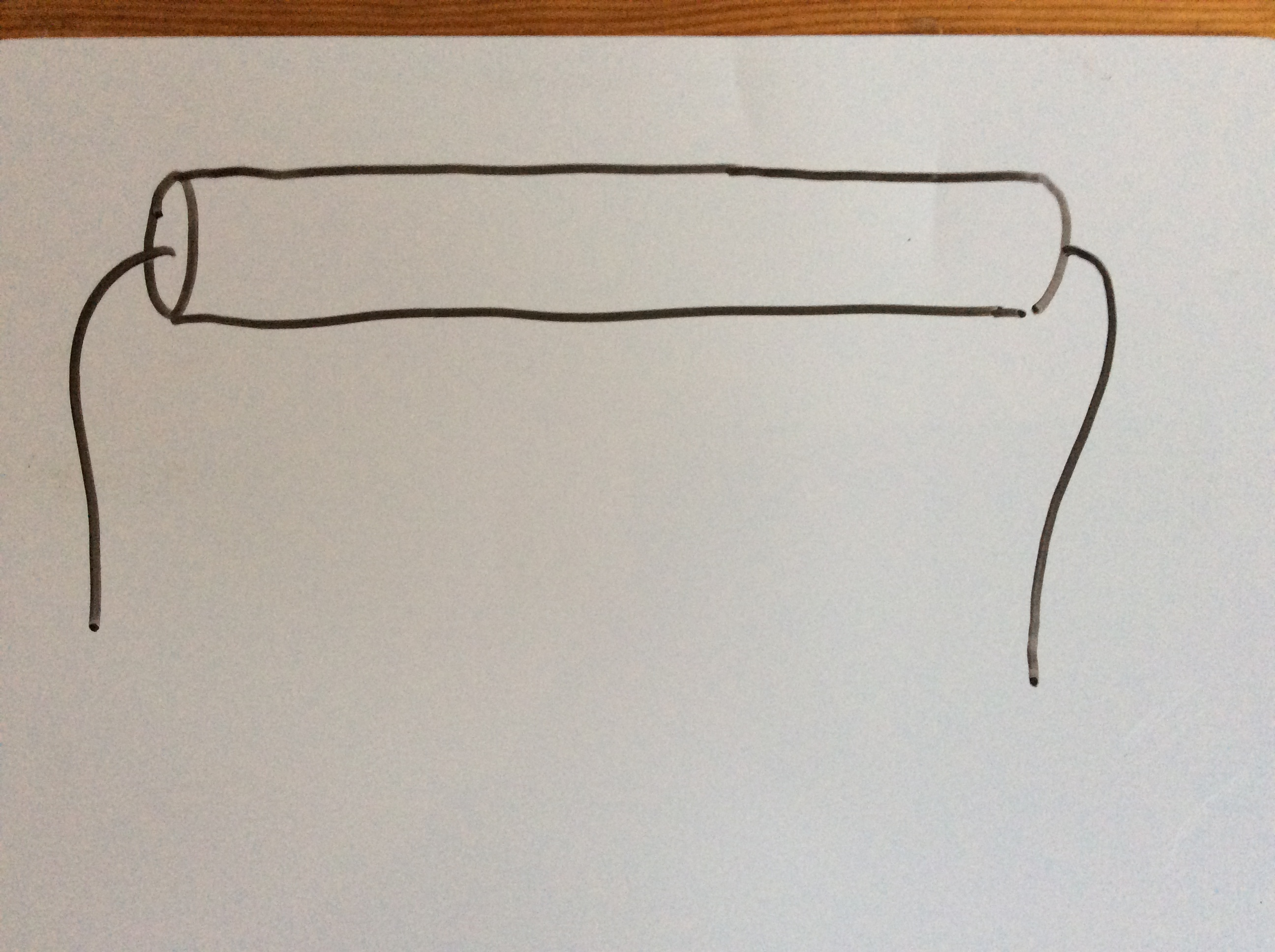• We can consider this wire to be the equivalent of a single resistor.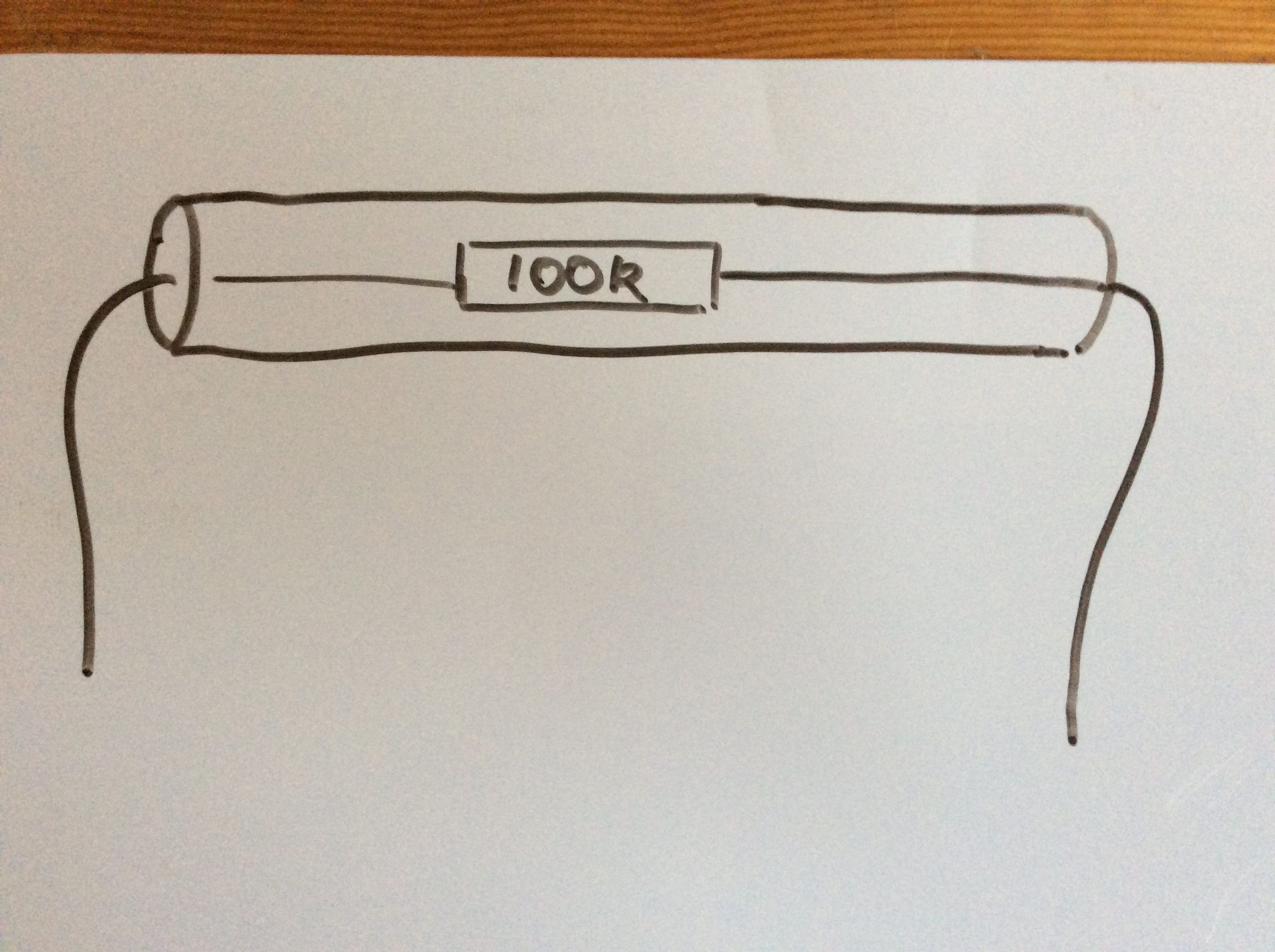• But we can place a third contact point midway along the wire. This now divides it up into two resistors.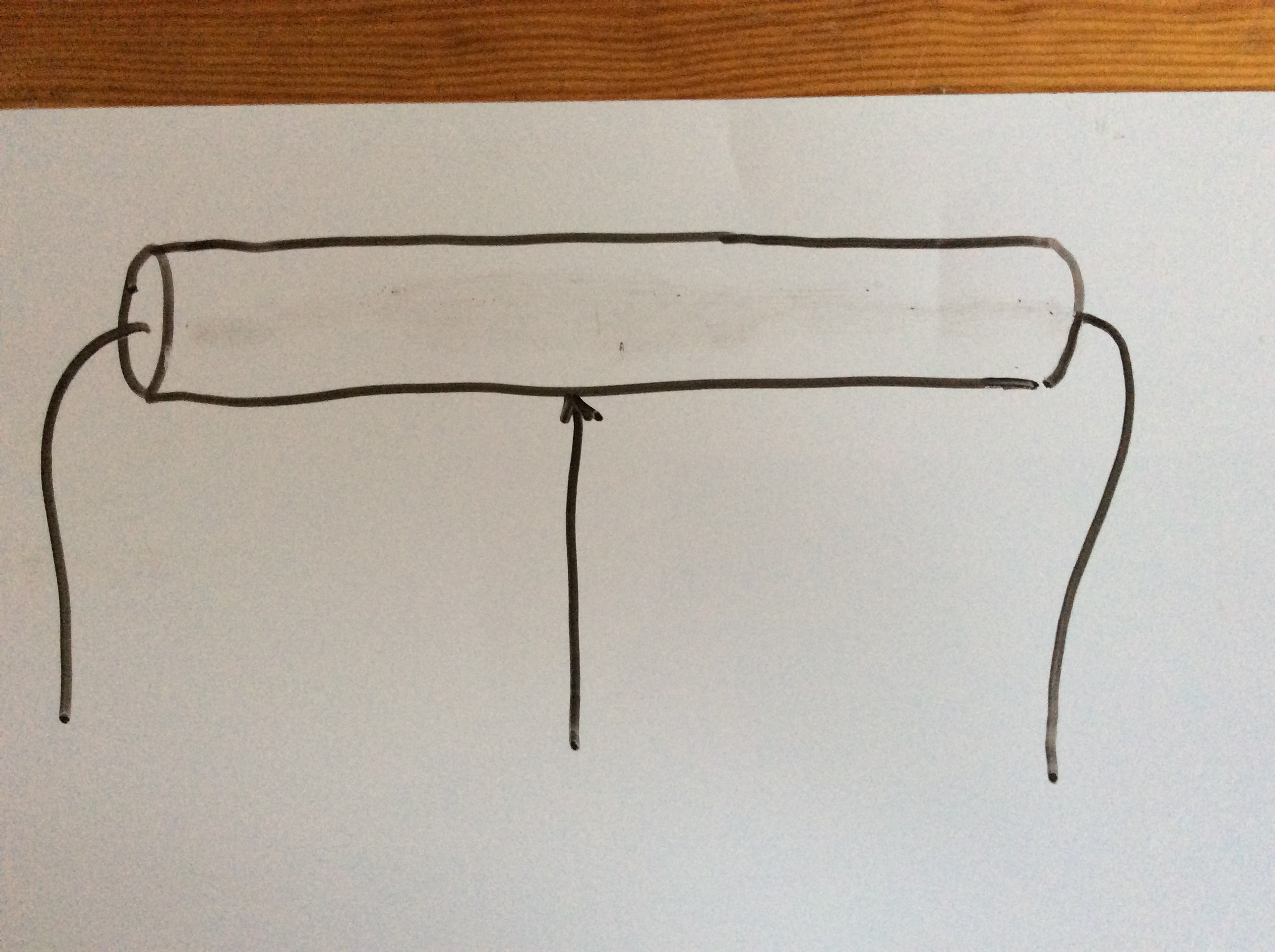• This then makes it the equivalent to two resistors.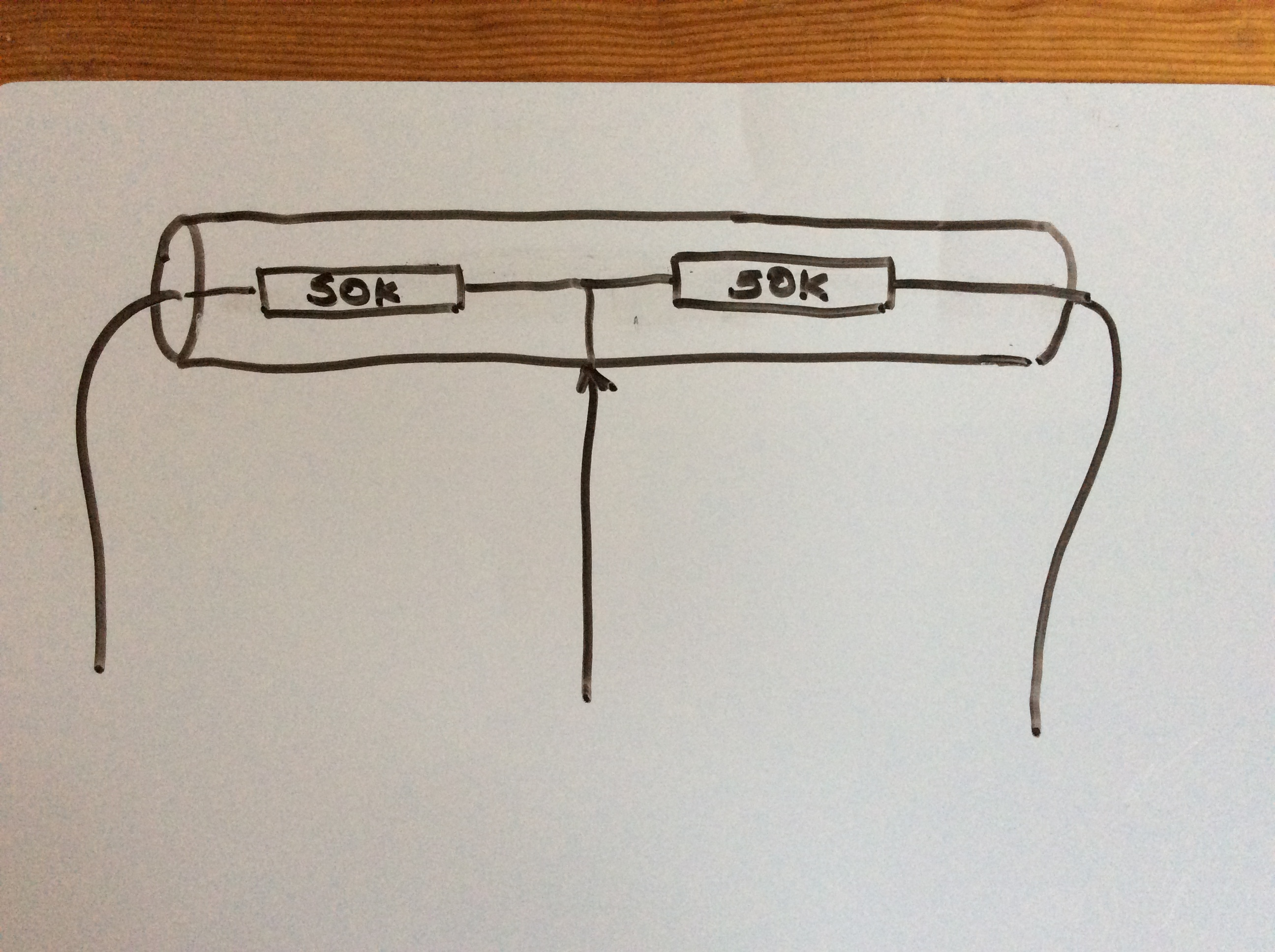• Now we can vary the size of the two resistors by moving the third contact point up and down the wire.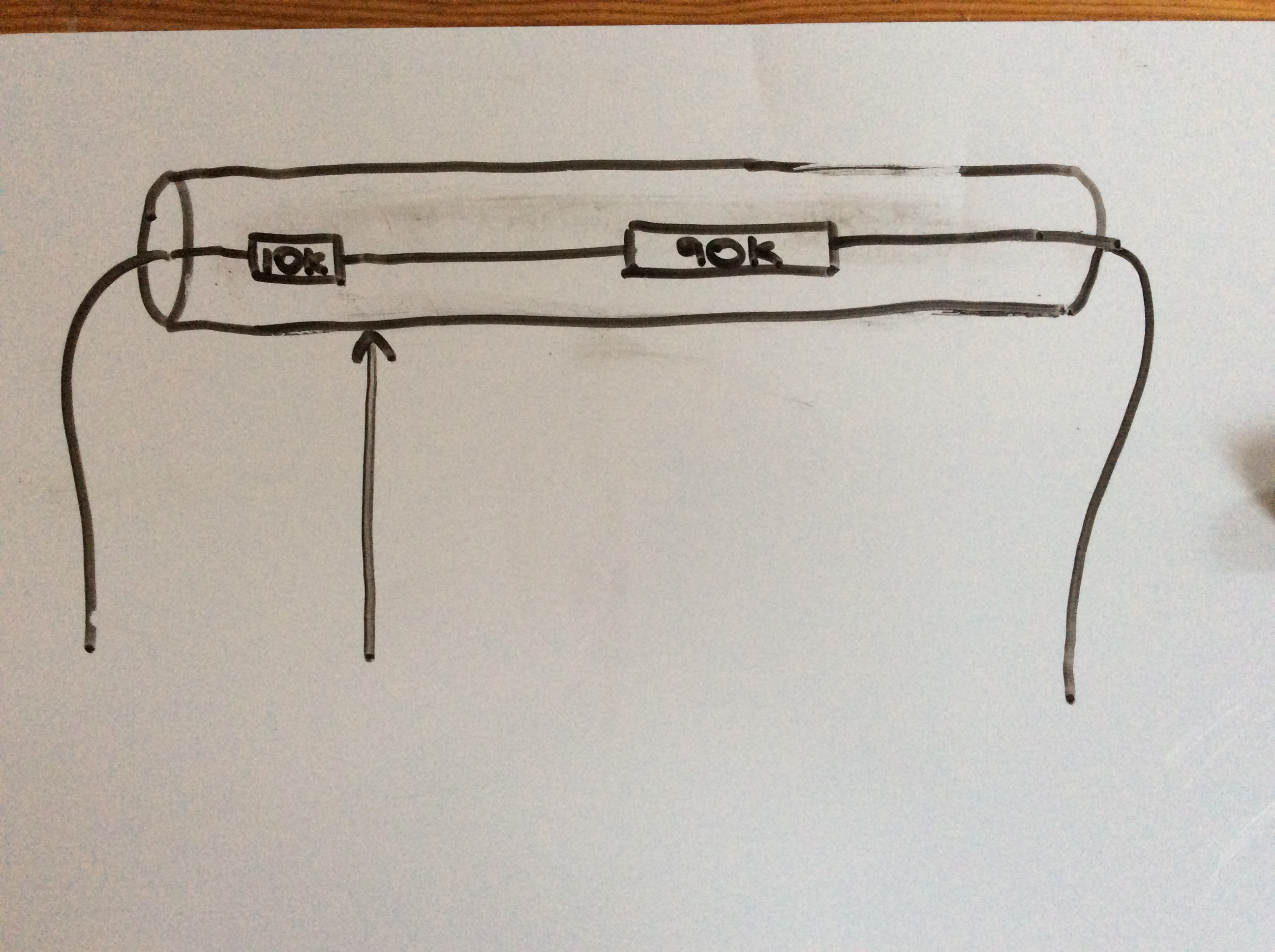• This is how a potentiometer works. A coil of wire is used and the third contact point is normally moved by rotating a dial.### Build It

• Let's place a potentiometer into a circuit.• Simulate the circuit and adjust the potentiometer to see how it effects the LED.